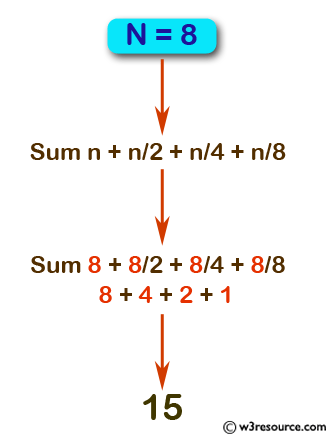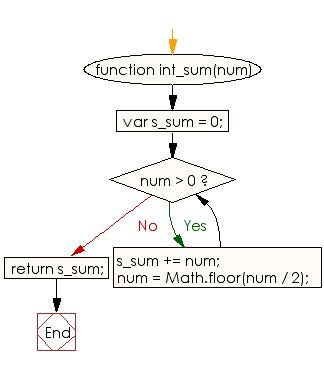# JavaScript: Calculate the sum of n + n/2 + n/4 + n/8 + ....

## JavaScript Basic: Exercise-113 with Solution

Write a JavaScript program to calculate the sum of n + n/2 + n/4 + n/8 + .... where n is a positive integer and all divisions are integer.

Pictorial Presentation:Sample Solution:

HTML Code:

``````<!DOCTYPE html>
<html>
<meta charset="utf-8">
<meta name="viewport" content="width=device-width">
<title> Calculate the sum of n + n/2 + n/4 + n/8 + ....</title>
<body>

</body>
</html>
```
```

JavaScript Code:

``````function int_sum(num) {
var s_sum = 0;
while (num > 0) {
s_sum += num;
num = Math.floor(num / 2);
}
return s_sum;
}
console.log(int_sum(8))
console.log(int_sum(9))
console.log(int_sum(26))
``````

Sample Output:

```15
16
49
```

Flowchart:ES6 Version:

``````function int_sum(num) {
let s_sum = 0;
while (num > 0) {
s_sum += num;
num = Math.floor(num / 2);
}
return s_sum;
}
console.log(int_sum(8))
console.log(int_sum(9))
console.log(int_sum(26))
``````

Live Demo:

See the Pen javascript-basic-exercise-113 by w3resource (@w3resource) on CodePen.

What is the difficulty level of this exercise?

Test your Programming skills with w3resource's quiz.

﻿

## JavaScript: Tips of the Day

Checks if a string is an anagram of another string (case-insensitive, ignores spaces, punctuation and special characters)

Example:

```const isAnagram = (str1, str2) => {
const normalize = str =>
str
.toLowerCase()
.replace(/[^a-z0-9]/gi, '')
.split('')
.sort()
.join('');
return normalize(str1) === normalize(str2);
};
console.log(isAnagram('iceman', 'cinema')); // true
```

Output:

```true
```